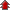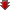Home Board Paper Solutions Text Book Solutions Articles NCERT Exemplar Solutions

# Chemical Kinetics### Exercise 1

•  Q1 For the reaction R → P, the concentration of a reactant changes from 0.03 M to 0.02 M in 25 minutes. Calculate the average rate of reaction using units of time both in minutes and seconds.">For the reaction R → P, the concentration of a reactant changes from 0.03 M to 0.02 M in 25 minutes. Calculate the average rate of reaction using units of time both in minutes and seconds. Q2 In a reaction, 2A → Products, the concentration of A decreases from 0.5 mol L-1 to 0.4 mol L-1 in 10 minutes. Calculate the rate during this interval?">In a reaction, 2A → Products, the concentration of A decreases from 0.5 mol L-1 to 0.4 mol L-1 in 10 minutes. Calculate the rate during this interval? Q3 For a reaction, A + B → Product; the rate law is given by,  r  = k [A]½ [B]2. What is the order of the reaction? Q4 The conversion of molecules X to Y follows second order kinetics. If concentration of X is increased to three times how will it affect the rate of formation of Y? Q5 A first order reaction has a rate constant 1.15 10-3s-1. How long will 5 g of this reactant take to reduce to 3 g? Q6 Time required to decompose SO2Cl2to half of its initial amount is 60 minutes. If the decomposition is a first order reaction, calculate the rate constant of the reaction. Q7 What will be the effect of temperature on rate constant? Q8 The rate of the chemical reaction doubles for an increase of 10 K in absolute temperature from 298 K. Calculate Ea. Q9 The activation energy for the reaction 2HI(g) → H2 + I2(g) is 209.5 kJ mol-1 at 581 K. Calculate the fraction of molecules of reactants having energy equal to or greater than activation energy?">The activation energy for the reaction 2HI(g) → H2 + I2(g) is 209.5 kJ mol-1 at 581 K. Calculate the fraction of molecules of reactants having energy equal to or greater than activation energy?## Recently Viewed Questions of Class 12th chemistry

• NCERT Chapter